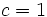# Schur multiplier of abelian group is its exterior square

Suppose$G$ is an abelian group. The Schur multiplier of$G$, denoted$M(G)$, which is the same as the second homology group for trivial group action$H_2(G;\mathbb{Z})$ is isomorphic to the group$\bigwedge^2 G$, defined as the exterior square of$G$ viewed as a$\mathbb{Z}$-module.
nilpotent multiplier instead of Schur multiplier nilpotent multiplier of abelian group is graded component of free Lie ring The Schur multiplier is the$c$-nilpotent multiplier for$c = 1$. Thus, it equals the$(c+1)^{th}$ graded component of the free Lie ring. In Lie theory, the relations in degree two are generated by the alternating condition, so the graded component is precisely the exterior square.